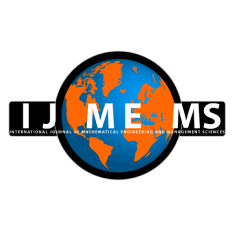### International Journal of Mathematical, Engineering and Management Sciences

#### ISSN: 2455-7749 . Open Access

Exact Solutions of (2+1) Dimensional Cubic Klein-Gordon (cKG) Equation

#### Exact Solutions of (2+1) Dimensional Cubic Klein-Gordon (cKG) Equation

Lakhveer Kaur
Department of Mathematics, Jaypee Institute of Information Technology, Noida, U.P., India.

Kuldeep
Department of Mathematics, Jaypee Institute of Information Technology, Noida, U.P., India.

;
Accepted on June 22, 2022

Abstract

In current study, (2+1)-dimensional cubic Klein-Gordon (cKG) equation illustrating dislocation propagation in crystals as well as the behaviour of elementary particles is investigated to establish a variety of new analytic exact solitary wave solutions. Modified exponential expansion method has been implemented to unfold certain wave solutions of considered model. As a result, three sorts of solutions emerge in a fairly systematic manner in the shape of hyperbolic, trigonometric, and rational functions. The kink and periodic wave solitons are acquired and presented geometrically, some 3D plots are simulated and displayed to respond the dynamic behavior of these obtained solutions. In this work we have used symbolic package maxima to obtained our solutions. Our acquired solutions might be most helpful to analyze physical issues that arise from nonlinear complicated dynamical systems.

Keywords- (2+1) dimensional cubic Klein-Gordon (cKG) equation; Modified exponential expansion method; Exact solutions; Trigonometric functions; Hyperbolic functions.

Citation

Kaur, L., & Kuldee (2022). Exact Solutions of (2+1) Dimensional Cubic Klein-Gordon (cKG) Equation. International Journal of Mathematical, Engineering and Management Sciences, 7(5), 613-623. https://doi.org/10.33889/IJMEMS.2022.7.5.040.